# Intermediate Geometry : How to find the volume of a cylinder

## Example Questions

1 2 3 5 Next →

### Example Question #60 : Cylinders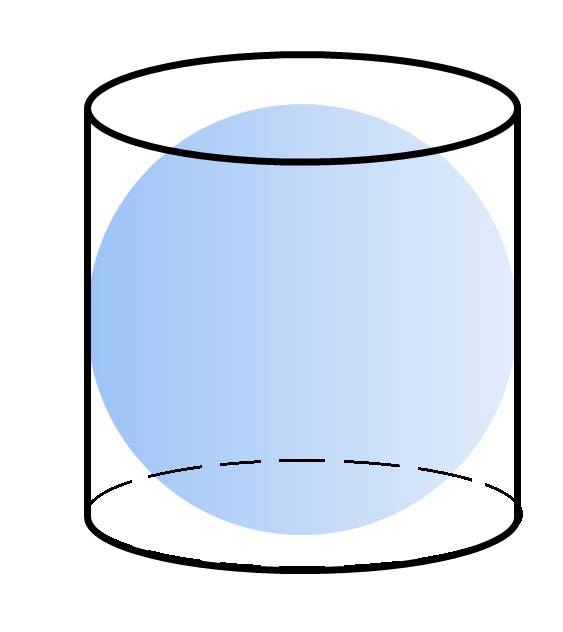The above diagram shows a sphere inscribed inside a cylinder.

The sphere has a volume of 100. Give the volume of the cylinder.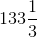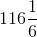Explanation:

Letbe the radius of the sphere. Then the radius of the base of the cylinder is also, and the height of the cylinder is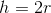.

The volume of the cylinder is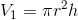,

which, after substituting, is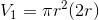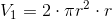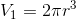The volume of the sphere is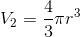Therefore, the ratio of the former to the latter is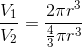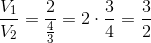and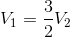That is, the volume of the cylinder istimes that of the sphere, so the volume of the cylinder is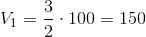.

### Example Question #1091 : Intermediate Geometry

During a snow storm,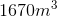of snow was collected. If the snow were put in a circular lot that had a radius of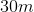, how high would the snow on the lot be?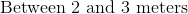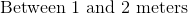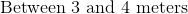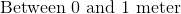Explanation:

Since we are putting the snow on a circular base, we are dealing with a cylinder. Notice that the radius of the cylinder and the volume of the cylinder are already given to you.

Recall how to find the volume of a cylinder: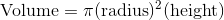Rearrange the equation to solve for the height: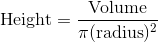Plug in the given volume and radius: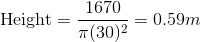The height is betweenandmeter.

1 2 3 5 Next →